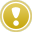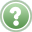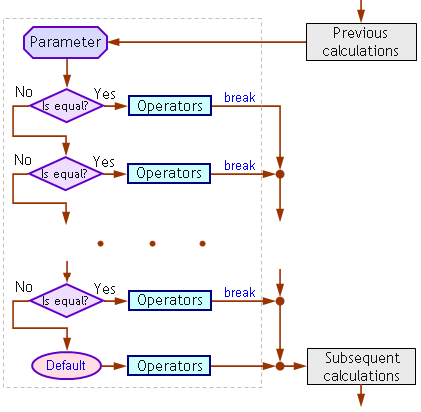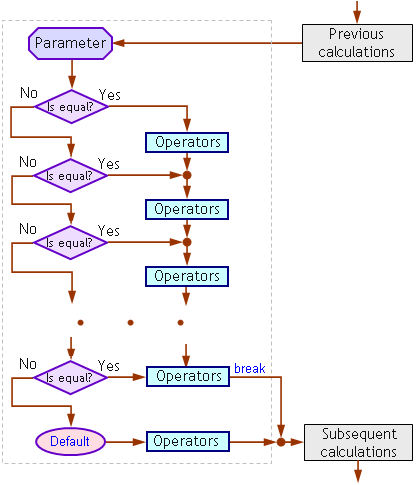English

MQL4 BookOperatorsOperator 'switch'

# Operator 'switch'

Some programs imply branching of their algorithm into several variations. In such cases, it is very convenient to use the operator 'switch', especially if there are tens or hundreds of variations, whereas the 'if' code becomes bloated if you use many nested operators.

## Format of the Operator 'switch'

The operator 'switch' consists of the header and the executable body. The header contains the name of the operator and an Expression enclosed in parentheses. The operator body contains one or several variations 'case' and one variation 'default'.

Each 'case' variation consists of the key word 'case', a Constant, ":" (colon), and operators. The amount of variations 'case' is not limited.

The variation 'default' consists of the key word 'default', ":" (colon), and operators. The variation 'default' is specified in the body of the operator 'switch' as the last, but it can also be located anywhere within the operator body or even be absent.

`   switch ( Expression )                           // Operator header       {                                          // Opening brace      case Constant: Operators                   // One of the 'case' variations       case Constant: Operators                   // One of the 'case' variations       ...      [default: Operators]                        // Variation without any parameter      }                                         // Closing brace`

The values of Expression and of Parameters can only be the values of int type. The Expression can be a constant, a variable, a function call, or an expression. Each variation 'case' can be marked by an integer constant, a character constant, or a constant expression. A constant expression cannot include variables or function calls.

## Execution Rule of the Operator 'switch'The program must pass the control to the first operator that follows the ":" (colon) of the variation 'case', the Constant value of which is the same as the value of the Expression, and then execute one by one all operators composing the body of the operator 'switch'. The condition of equality between the Expression and the Constant is tested in the direction from top to bottom and from left to right. The values of Constants in different variations 'case' must not be the same. The operator 'break' stops the execution of the operator 'switch' and passes the control to the operator that follows the operator 'switch'.

It is easy to see that the 'case Constant:' represents just a label, to which the control is passed. All operators that compose the body of the operator 'switch' start being executed from this label. If the program algorithm implies the execution of a group of operators that correspond with only one variation 'case', you must specify the operator 'break' as the last in the list of operators that correspond with only one variation 'case'. Let's consider the work of the operator 'switch' using some examples.

## Examples of the Operator 'switch'Problem 18. Compose a program where the following conditions are realized: If the price exceeds the predefined level, the program must inform the trader about it using a message where the excess is described in words (up to 10 points); in other cases, the program must inform that the price does not exceed the predefined level.

Below is the problem solution using the operator 'switch' (Expert Advisor pricealert.mq4):

`//-------------------------------------------------------------------------------------// pricealert.mq4// The code should be used for educational purpose only.//-------------------------------------------------------------------------------------int start()                                     // Special function 'start'  {   double Level=1.3200;                           // Preset price level   int Delta=NormalizeDouble((Bid-Level)Point,0); // Excess    if (Delta<=0)                                  // Price doesn't excess the level     {      Alert("The price is below the level");      // Message      return;                                     // Exit start()     }//-------------------------------------------------------------------------------------   switch(Delta)                                  // Header of the 'switch'     {                                            // Start of the 'switch' body      case 1 : Alert("Plus one point");     break;// Variations..      case 2 : Alert("Plus two points");    break;      case 3 : Alert("Plus three points");  break;      case 4 : Alert("Plus four points");   break;//Here are presented      case 5 : Alert("Plus five points");   break;//10 variations 'case',      case 6 : Alert("Plus six points");    break;//but, in general case,      case 7 : Alert("Plus seven points");  break;//the amount of variations 'case'      case 8 : Alert("Plus eight points");  break;//is unlimited      case 9 : Alert("Plus nine points");   break;      case 10: Alert("Plus ten points");    break;      default: Alert("More than ten points");     // It is not the same as the 'case'     }                                            // End of the 'switch' body//-------------------------------------------------------------------------------------   return;                                        // Exit start()  }//-------------------------------------------------------------------------------------`

In this problem solution, we use the operator 'switch', in which each variation 'case' uses the operator 'break'. Depending on the value of variable Delta, the control will be passed to one of variations 'case'. This results in that the operators corresponding with this variation are executed: function Alert() and the operator 'break'. The operator 'break' stops execution of the operator 'switch', i.e., it passes the control outside it, namely, to the operator 'return' that ends the operation of the special function start(). Thus, depending on the value of variable Delta, one of variations 'case' triggers, whereas other variations remain untouched.

The above program is an Expert Advisor, so it will be launched for execution at every tick and it will every time display the messages corresponding with the current situation. Of course, we should search a value for the Level possibly close to the current price of the symbol, the window, to which we are attaching this EA.Fig. 49. Block diagram of the operator 'switch' in EA pricealert.mq4.

In the block diagram above, we can clearly see that, due to the presence of the operator 'break' in each variation 'case', the control is passed outside the operator 'switch' after the operators of any variation 'case' have been executed. A similar principle of algorithm construction using the operator 'switch' is utilized in the file named stdlib.mq4 delivered within the client terminal (..\experts\libraries\stdlib.mq4).

Let's consider another problem that does not provide the usage of 'break' in each variation 'case'.Problem 19. There are 10 bars. Report the numbers of all bars starting with the nth one.

It is rather easy to code the solution of this problem (script barnumber.mq4):

`//------------------------------------------------------------------------------// barnumber.mq4// The code should be used for educational purpose only.//------------------------------------------------------------------------------int start()                             // Special function start()  {   int n = 3;                           // Preset number   Alert("Bar numbers starting from ", n,":");// It does not depend on n //------------------------------------------------------------------------------   switch (n)                           // Header of the operator 'switch'     {                                  // Start of the 'switch' body      case 1 : Alert("Bar 1");          // Variations..      case 2 : Alert("Bar 2");          case 3 : Alert("Bar 3");          case 4 : Alert("Bar 4");          // Here are 10 variations ..      case 5 : Alert("Bar 5");          // ..'case' presented, but, in general, ..      case 6 : Alert("Bar 6");          // ..the amount of variations..      case 7 : Alert("Bar 7");          // ..'case' is unlimited        case 8 : Alert("Bar 8");      case 9 : Alert("Bar 9");      case 10: Alert("Bar 10");break;          default: Alert("Wrong number entered");// It is not the same as the 'case'     }                                  // End of the 'switch' body//-------------------------------------------------------------------------------   return;                              // Operator to exit start()  }//-------------------------------------------------------------------------------`

In the operator 'switch', the program will search in the variations 'case', until it detects that the Expression is equal to the Constant. When the value of the Expression (in our case, it is integer 3) is equal to one of the Constants (here, it is case 3), all operators that follows the colon (case 3:) will be executed, namely: the operator of function call Alert("Bar 3"), those following Alert("Bar 4"), Alert("Bar 5"), etc., until it comes to the operator 'break' that terminates the operation of the operator 'switch'.

If the value of the Expression does not coincide with any of the Constants, the control is passed to the operator that corresponds to the variation 'default':Fig. 50. Block diagram of the operator 'switch' in script barnumber.mq4.

Unlike the algorithm realized in the preceding program, in this case (Fig. 50), we are not using the operator 'break' in each variation 'case'. So, if the value of the Expression is equal to the value of one of the Constants, all operators will be executed starting with the operators of the corresponding variation 'case'. We also use the operator 'break' in the last variation 'case' for another purpose: to prevent the operators corresponding with the variation 'default' from executing. If there is no value equal to the Expression among the values of the Constants, the control will be passed to the operator that corresponds with the 'default' label.

Thus, if the value of the preset variable n is within the range of values from 1 to 10, the numbers of all bars will be printed starting with the nth one. If the value of n is beyond the above range, the program will inform the user about no matches.Please note: It is not necessary that the Constants of the variations 'case' are arranged in your program in order of magnitude. The order of how the variations 'case' with the corresponding Constants follow each other is determined by the needs of the algorithm of your program.Operator 'continue'Function Call